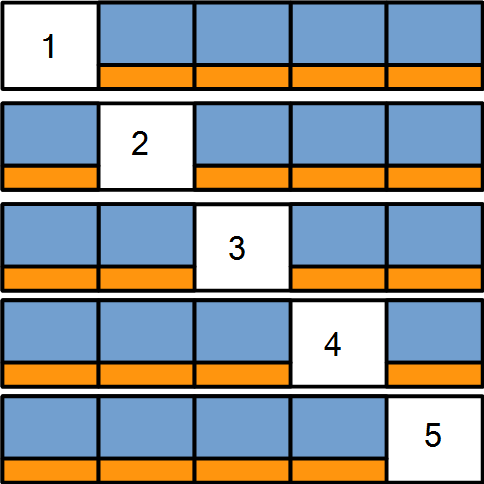# K-Fold Cross-Validation

In this post, we are going to look at k-fold cross-validation and its use in evaluating models in machine learning.

K-fold cross-validation is used for determining the performance of statisticalmodels. How it works is the data is divided into a predetermined number of folds (called ‘k’). One fold is used to determine the model estimates and the other folds are used for evaluating. This is done k times and the results are average based on a statistic such as kappa to see how the model performs.

In our example, we are going to review a model we made using the C5.0 algorithm. In that post, we were trying to predict gender based on several other features.

First, we need to load several packages into R as well as the dataset we are going to use. All of this is shared in the code below

``library(caret);library(C50);library(irr);library(Ecdat)``
``data("Wages1")``

We now need to set the seed. This is important for allowing us to reproduce the results. Every time a k-fold is performed the results can be slightly different but setting the seed prevents this. The code is as follows

``set.seed(1)``

We will now create are folds. How many folds to create is up to the researcher. For us, we are going to create ten folds. What this means is that R will divide our sample into ten equal parts. To do this we use the “createFolds” function from the “caret” package. After creating the folds, we will view the results using the “str” function which will tell us how many examples are in each fold. Below is the code to complete this.

``````folds<-createFolds(Wages1\$sex, k=10)
str(folds)``````
``````## List of 10
##  \$ Fold01: int [1:328] 8 13 18 37 39 57 61 67 78 90 ...
##  \$ Fold02: int [1:329] 5 27 47 48 62 72 76 79 85 93 ...
##  \$ Fold03: int [1:330] 2 10 11 31 32 34 36 64 65 77 ...
##  \$ Fold04: int [1:330] 19 24 40 45 55 58 81 96 99 102 ...
##  \$ Fold05: int [1:329] 6 14 28 30 33 84 88 91 95 97 ...
##  \$ Fold06: int [1:330] 4 15 16 38 43 52 53 54 56 63 ...
##  \$ Fold07: int [1:330] 1 3 12 22 29 50 66 73 75 82 ...
##  \$ Fold08: int [1:330] 7 21 23 25 26 46 51 59 60 83 ...
##  \$ Fold09: int [1:329] 9 20 35 44 49 68 74 94 100 105 ...
##  \$ Fold10: int [1:329] 17 41 42 71 101 107 117 165 183 184 ...``````

As you can see, we normally have about 330 examples per fold.

In order to get the results that we need. We have to take fold 1 to make the model and fold 2-10 to evaluate it. We repeat this process until every combination possible is used. First, fold 1 is used and 2-10 are the test data, then fold 2 is used and then folds 1, 3-10 are the test data etc.  Manually coding this would take a great deal of time. To get around this we will use the “lapply” function.

Using “lapply” we will create a function that takes “x” (one of our folds) and makes it the “training set” shown here as “Wages1_train”. Next, we assigned the rest of the folds to be the “test” (Wages1_test) set as depicted with the “-x”. The next two lines of code should look familiar as it is the code for developing a decision tree. The “Wages_actual” are the actual labels for gender in the “Wages_1” testing set.

The “kappa2” function is new and it comes from the “irr” package. The kappa statistic is a measurement of the accuracy of a  model while taking into account chance. The closer the value is to 1 the better. Below is the code for what has been discussed.

``````results<-lapply(folds, function(x) {
Wages1_train<-Wages1[x, ]
Wages1_test<-Wages1[-x, ]
Wages1_model<-C5.0(sex~.,data=Wages1)
Wages1_pred<-predict(Wages1_model, Wages1_test)
Wages1_actual<-Wages1_test\$sex
Wages1_kappa<-kappa2(data.frame(Wages1_actual, Wages1_pred))\$value
return(Wages1_kappa)
})``````

To get our results, we will use the “str” function again to display them. This will tell us the kappa for each fold. To really see how our model does we need to calculate the mean kappa of the ten models. This is done with the “unlist” and “mean” function as shown below

``str(results)``
``````## List of 10
##  \$ Fold01: num 0.205
##  \$ Fold02: num 0.186
##  \$ Fold03: num 0.19
##  \$ Fold04: num 0.193
##  \$ Fold05: num 0.202
##  \$ Fold06: num 0.208
##  \$ Fold07: num 0.196
##  \$ Fold08: num 0.202
##  \$ Fold09: num 0.194
##  \$ Fold10: num 0.204``````
``mean(unlist(results))``
``##  0.1978915``

The final mean kappa was 0.19 which is really poor. It indicates that the model is no better at predicting then chance alone. However, for illustrative purposes, we now understand how to perform a k-fold cross-validation